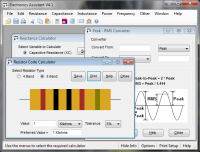# Power Calculator

These calculators perform calculations associated with power (wattage). The bottom one calculates power dissipated in a resistor given the current flowing through it.

#### Calculate Power from Voltage & Current (P = V * I):

 Enter Voltage: Volts Millivolts Microvolts Enter Current: Amps Milliamps Microamps Power = ?

#### Calculate Voltage from Power & Current (V = P / I):

 Enter Power: Watts Milliwatts Microwatts Enter Current: Amps Milliamps Microamps Voltage = ?

#### Calculate Current from Power & Voltage (I = P / V):

 Enter Power: Watts Milliwatts Microwatts Enter Voltage: Volts Millivolts Microvolts Current = ?

#### Calculate Power Dissipated in a Resistor (P = I2 * R):

 Enter Current: Amps Milliamps Microamps Enter Resistance: Ohms Kilohms Megohms Power = ?

#### Formulae:

The formula for calculating power in DC circuits is:

P = V * I, where P is power in Watts, V is voltage in Volts and I is current in Amps

This can be re-arranged to give:

V = P / I
I = P / V

Combining this equation with Ohms Law gives the power dissipated in a resistor:

P = V * I
V = I * R (Ohms Law)
Combining these gives P = I * I * R
or P= I2 * R

See here for more details.

# If you found this calulator useful you should try Electronics Assistant!• All the online calculators and more in a stand-alone application
• Converts Resistor & Inductor colour codes, calculates LED series resistors, capacitance units, series / parallel resistors & capacitors, frequency, reactance & more
• Calculation of nearest preferred resistor values with a choice of 5 series from E12 to E192
• Print & save calculation results